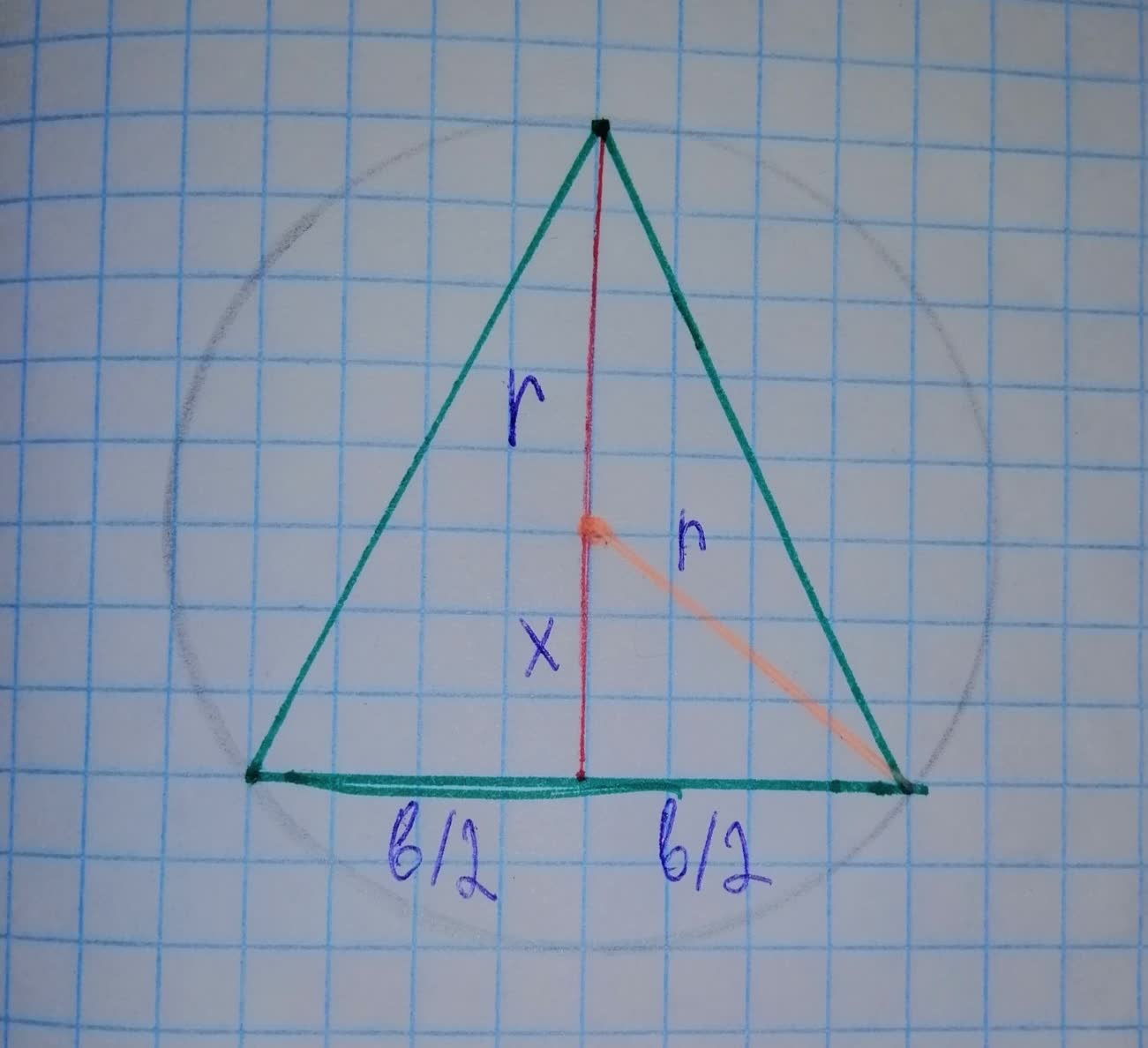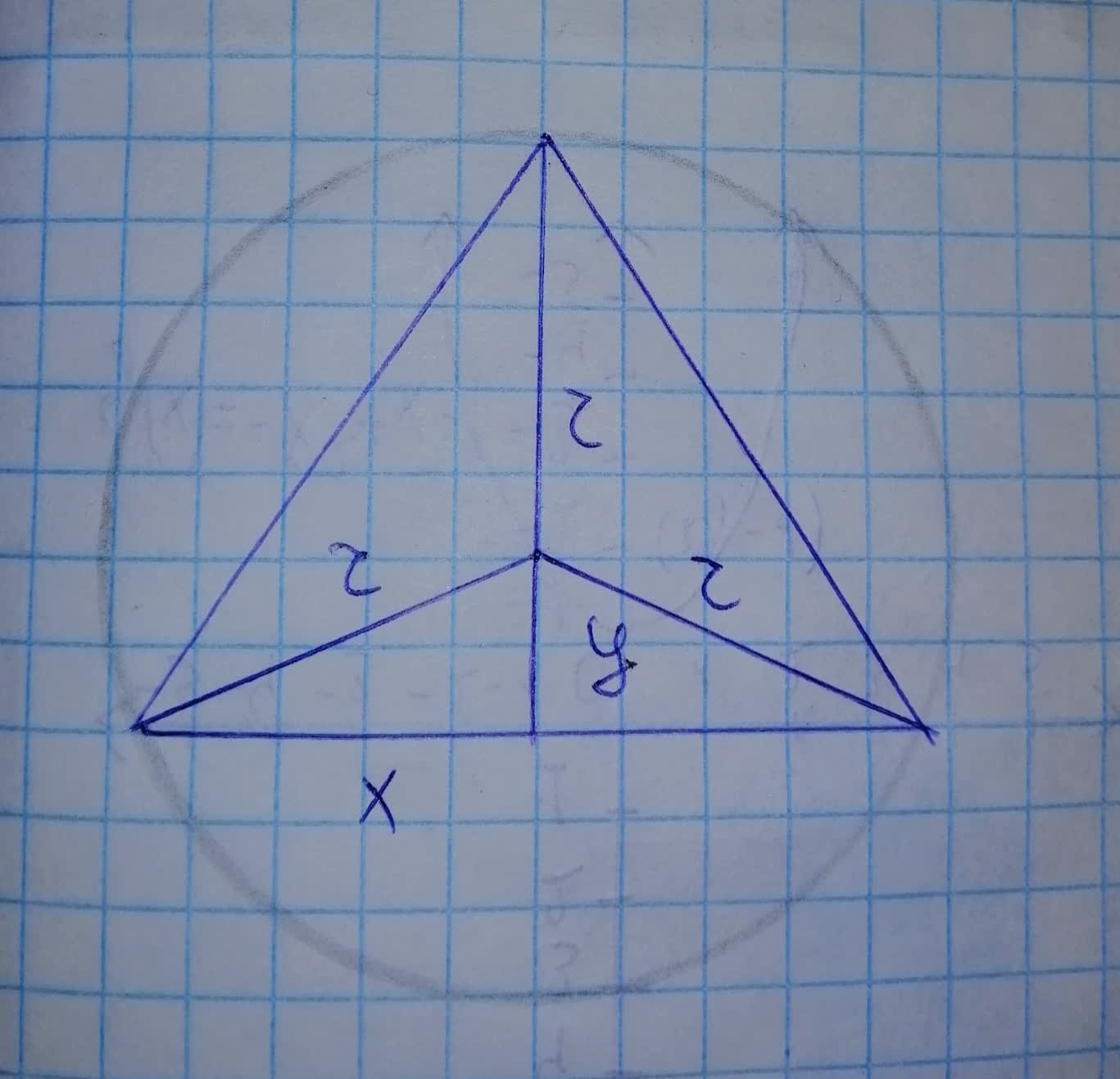# Find the dimensions of the isosceles triangle of largest area that can be inscriIMLOG10ct 2021-11-20 Answered
Find the dimensions of the isosceles triangle of largest area that can be inscribed in a circle of radius r.
You can still ask an expert for help

• Questions are typically answered in as fast as 30 minutes

Solve your problem for the price of one coffee

• Math expert for every subject
• Pay only if we can solve itWasither1957

Step 1
From the figure, by Pythagoras theorem we can write
$\frac{b}{2}=\sqrt{{r}^{2}-{x}^{2}}$
$b=2\sqrt{{r}^{2}-{x}^{2}}\to \left(1\right)$Step 2
Area of Triangle $=\frac{1}{2}$ (Base)(Height)
$A=\frac{1}{2}\left(b\right)\left(r+x\right)$
Use Eqn(1) to substitute the value of b
$A=\frac{1}{2}\left(2\sqrt{{r}^{2}-{x}^{2}}\right)\left(r+x\right)$
$A\left(x\right)=\left(r+x\right)\sqrt{{r}^{2}-{x}^{2}}$
Step 3
Differentiate $A\left(x\right)$
${A}^{\prime }\left(x\right)=\frac{d\left[\left(r+x\right)\sqrt{{r}^{2}-{x}^{2}}\right]}{dx}$
Use Product Rule
${A}^{\prime }\left(x\right)=\sqrt{{r}^{2}-{x}^{2}}\cdot \frac{d\left[r+x\right]}{dx}+\left(r+x\right)\cdot \frac{d\left[\sqrt{{r}^{2}-{x}^{2}}\right]}{dx}$
${A}^{\prime }\left(x\right)=\sqrt{{r}^{2}-{x}^{2}}\cdot 1+\left(r+x\right)\cdot \frac{d\left[\sqrt{{r}^{2}-{x}^{2}}\right]}{d\left({r}^{2}-{x}^{2}\right)}×\frac{d\left({r}^{2}-{x}^{2}\right)}{dx}$
${A}^{\prime }\left(x\right)=\sqrt{{r}^{2}-{x}^{2}}+\left(r+x\right)\cdot \frac{1}{2\sqrt{{r}^{2}-{x}^{2}}}×\left(-2x\right)$
${A}^{\prime }\left(x\right)=\sqrt{{r}^{2}-{x}^{2}}-\frac{rx+{x}^{2}}{\sqrt{{r}^{2}-{x}^{2}}}$
Step 4
Solve for ${A}^{\prime }\left(x\right)=0$
$\sqrt{{r}^{2}-{x}^{2}}-\frac{rx+{x}^{2}}{\sqrt{{r}^{2}-{x}^{2}}}=0$Elizabeth Witte

Step 1
The equation of circle will be
${x}^{2}+{y}^{2}={r}^{2}$Step 2
Now, area of triangle
$A=\frac{1}{2}\left(2x\right)\left(r+y\right)$
$=x\left(r+y\right)$
$A\left(y\right)=\sqrt{{r}^{2}-{y}^{2}}\left(r+y\right)$
triangle derivative
${A}^{\prime }\left(y\right)=\sqrt{{r}^{2}-{y}^{2}}+\left(r+y\right)\cdot \frac{-2y}{2\sqrt{{r}^{2}-{y}^{2}}}$
$=\frac{{r}^{2}-ry-2{y}^{2}}{\sqrt{{r}^{2}-{y}^{2}}}$
equating to zero
$\frac{{r}^{2}-ry-2{y}^{2}}{\sqrt{{r}^{2}-{y}^{2}}}=0$
${r}^{2}-ry-2{y}^{2}=0$

rejecting, $y=-r$
Therefore, we check to see $y=\frac{r}{2}$ is maximum
${A}^{\prime }\left(y\right)>0$ for $y<\frac{r}{2}$ and ${A}^{\prime }\left(y\right)<0$ for $y>\frac{r}{2}$
$\therefore y=\frac{r}{2}$ is maximum
${x}^{2}+{\left(\frac{r}{2}\right)}^{2}={r}^{2}⇒x=\frac{\sqrt{3}}{2}r$
Dimension of triangle with maximum area is base
$=2x=2\cdot \frac{\sqrt{3}}{2}r=\sqrt{3}r$
$h=r+y=r+\frac{r}{2}=\frac{3}{2}r$